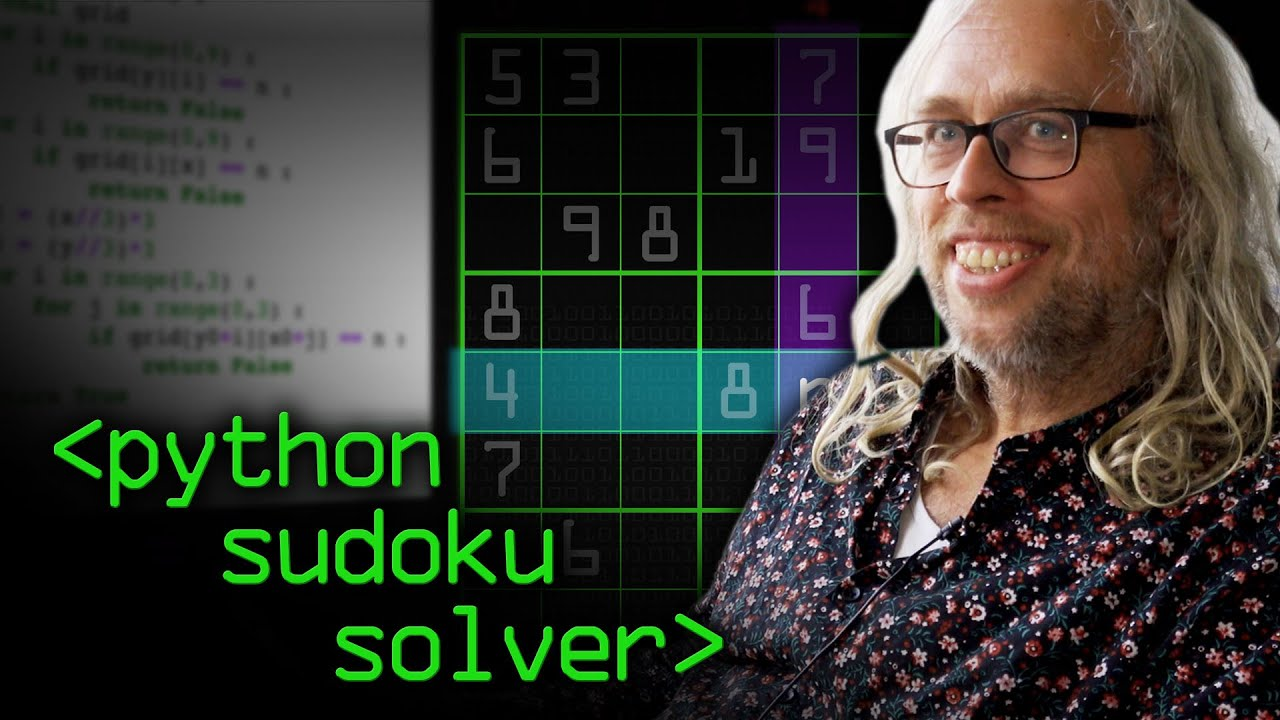# RecursionPython

#### Recursion and Is It Better Than For Loops?

In this tutorial, learn about recursion and how we can use it to divide and conquer! 💪💪💪 TIME STAMPS: 00:00 – Intro 00:15 – What is Recursion 01:03 – Python Recursive Function Code Example 02:43 – Fibonacci Sequence 03:49 – Fibonacci Recursion Code Example 05:21 – Fibonacci Iteration Code Example 06:56 – Is Python Recursion […]Developer

#### 5 Simple Steps for Solving Any Recursive Problem

This video we takes a look at one of the more challenging computer science concepts: Recursion. Learn the 5 simple steps to help you solve challenging recursive problems and show you 3 specific examples, each progressively more difficult than the last.Python

#### Python Sudoku Solver

Professor Thorsten Altenkirch demonstrates a recursive Sudoku solver.Python

#### Python Tutorial on Recursion, the Fibonacci Sequence and Memoization

Socratica explores recursion by writing a function to generate the terms of the Fibonacci sequence. We will use a technique called “memoization” to make the function fast. We’ll first implement our own caching, but then we will use Python’s builtin memoization tool: the lru_cache decorator.